Awschalom Group
g-Tensor Modulation Resonance

Previously demonstrated electrical control of spin coherence leads to a hope that dynamic and three dimensional control over electron spin could be achieved in a solid state device. Such manipulations are conventionally achieved through electron spin resonance techniques, which requires microwave magnetic fields. We demonstrate that if the g-factor is anisotropic, electron spins can be electrically manipulated through a new class of resonance, in a manner similar to electron spin resonance.

Generally, the g-factor can be described as a tensor. The interaction of electron spins with applied magnetic filed can be written in this Hamiltonian: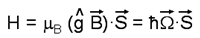Considering the case where a magnetic field B0 is applied at 45 degrees in between the x-axis and the z-axis, one can see that the spin precession axis depends on the ratio of the two components of the g-tensor.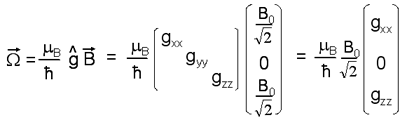This implies that it is possible to change the direction of the precession axis by changing the g-tensor with voltage as was done in previous work. Modulating the spin precession axis at the resonant frequency is analogous to the case of ESR, and should result in the tipping of the spin vector, i.e., g-tensor modulation resonance (g-TMR).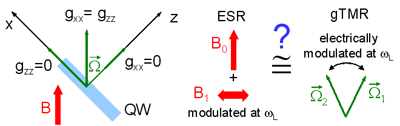There are two requirements in order for this concept to work. First, we have to be able to modulate the g-tensor at GHz frequencies, because that is the frequency at which the electron spins precess. Next, the g-tensor must be anisotropic, otherwise the precession axis will not change its direction.

We confirm the first point by applying a time dependent voltage V(t)=V0+V1sin(ωt+Φ). Using time resolved Kerr rotation (TRKR), we investigate the spin dynamics at 5K.In panel A, TRKR scan with V0=0 and Φ=0 is shown in blue, and the applied voltage is shown in red. As expected, the spin precession is frequency modulated, and its phase and average frequency can be tuned independently (panel B and C, respectively).

Next, the anisotropy of the g-tensor is demonstrated by performing TRKR in two geometries (at 0 an 45 degrees).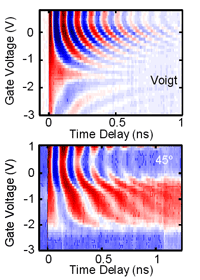To detect g-TMR, we place the sample at 45 degrees to the magnetic field, fix the delay to 3ns and fix the microwave phase and frequency. The color plot shows microwave induced TRKR as a function of magnetic field and DC voltage. As the magnitude of precession vector changes with DC bias, the resonance position moves along with it. A typical line cut along magnetic field is shown below.The final check that this resonance is due to the proposed mechanism of g-TMR is done by looking at the angle dependence. According to our theoretical model, g-TMR should diminish when the magnetic field is applied along one of the principal axes. The figure shows good agreement with the model (solid line) while the ESR model (dotted line) fails to explain the data.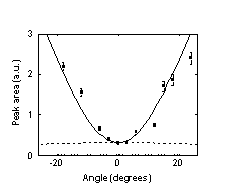To learn more about our studies, please refer to "Gigahertz electron spins manipulation using voltage controlled g-tensor modulation", Y. Kato, R. C. Myers, D. Driscol, A. C. Gossard, J. Levy, and D. D. Awschalom, Science 299, 1201 (2003) Previous Research Index Related Publications Next